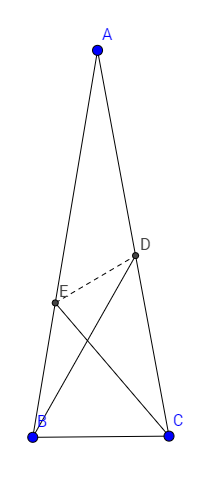# Are you too tired to chase angles?

Geometry Level 3Let ABC be an isosceles triangle $AB=AC$ with $\angle BAC = 20^{\circ}$. Point $D$ is on side $AC$ such that $\angle DBC = 60^{\circ}$. Point $E$ is on side $AB$ such that $\angle ECB = 50^{\circ}$. Find the measure of $\angle EDB$ in degrees.

×